# 图像识别初步

Posted by jjx on January 4, 2017

• 模式与模式识别的基本概念
• 过度拟合
• 最小距离分类器
• 基于相关的模板匹配
• 本章的典型案例分析
• 基于最小距离分类器的鸾尾属植物分类
• 基于相关技术的图像模式匹配

### 模式识别概述

#### 关键概念

• 模式类(pattern class): 指共享一组共同属性（或特征）的模式集合， 通常具有相同的来源。
• 特征(feature): 指一种模式区别于另一种模式的相应（本质）特点或特性， 是通过测量和或处理能够抽取的数据
• 噪声(noise): 指与模式处理（特征抽取中的误差）和（或）训练样本联合的失真．它对系统的分类能力（如识别）产生影响．
• 分类／识别(classfication/Recognition): 指根据特征将模式分配给不同的模式类， 识别出模式的类别的过程．
• 分类器(classifier): 指为了实现分类而建立起来某种计算模型，它以模式特征为输入，输出该模式所属的类别信息．
• 训练样本(training sample): 指一些类别信息已知的样本，通常使用它们来训练分类器．
• 训练集合(training set) : 训练样本所组成的集合．
• 训练学习(training learning): 指根据训练样本集合， “教授” 识别系统如何将输入矢量映射为输出矢量的过程．
• 测试样本(testing sample): 指一些类别信息对于分类器未知（不提供给分类器其类别信息）的样本， 通常使用它们来测试分类器的性能．
• 测试集合C testing set): 指测试样本所组成的集合． 当测试集合与训练集合没有交集时， 称为独立的测试集．
• 测试(testing): 指将测试样本作为输入送入已训练好的分类器，得到分类结果并对分类正确率进行统计的过程．
• 识别率(accuracy): 指对于某一样本集合而言，经分类器识别正确的样本占总样本数的比例。
• 泛化精度(generalization accuracy): 指分类器在独立于训练样本的测试集合上的识别率．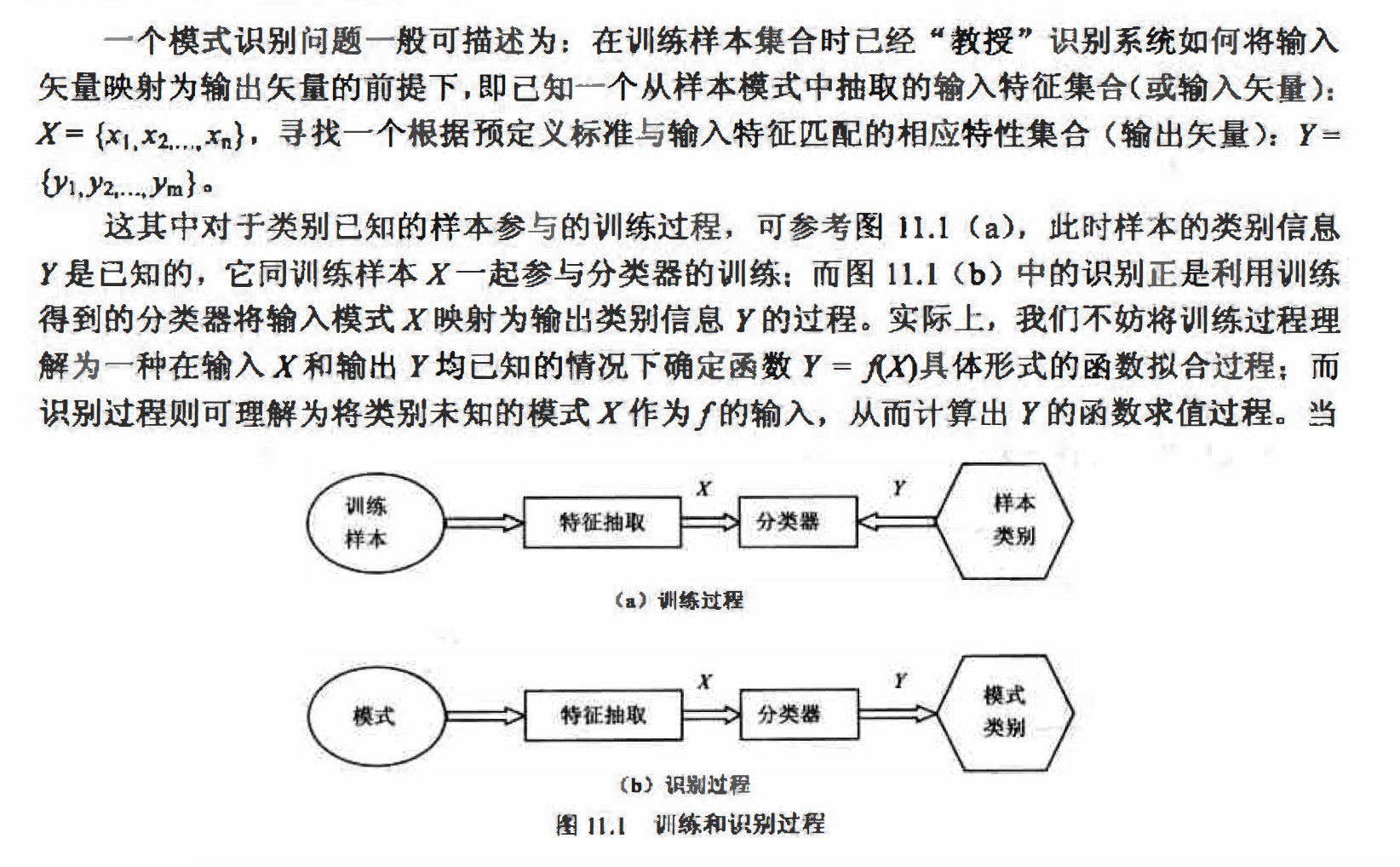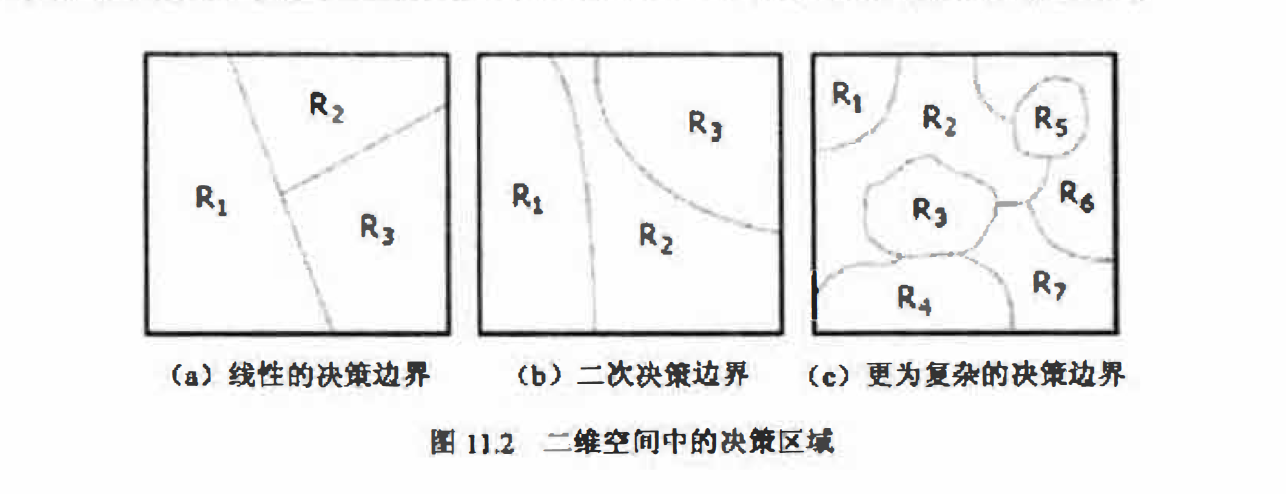#### 过度拟合(Overlit)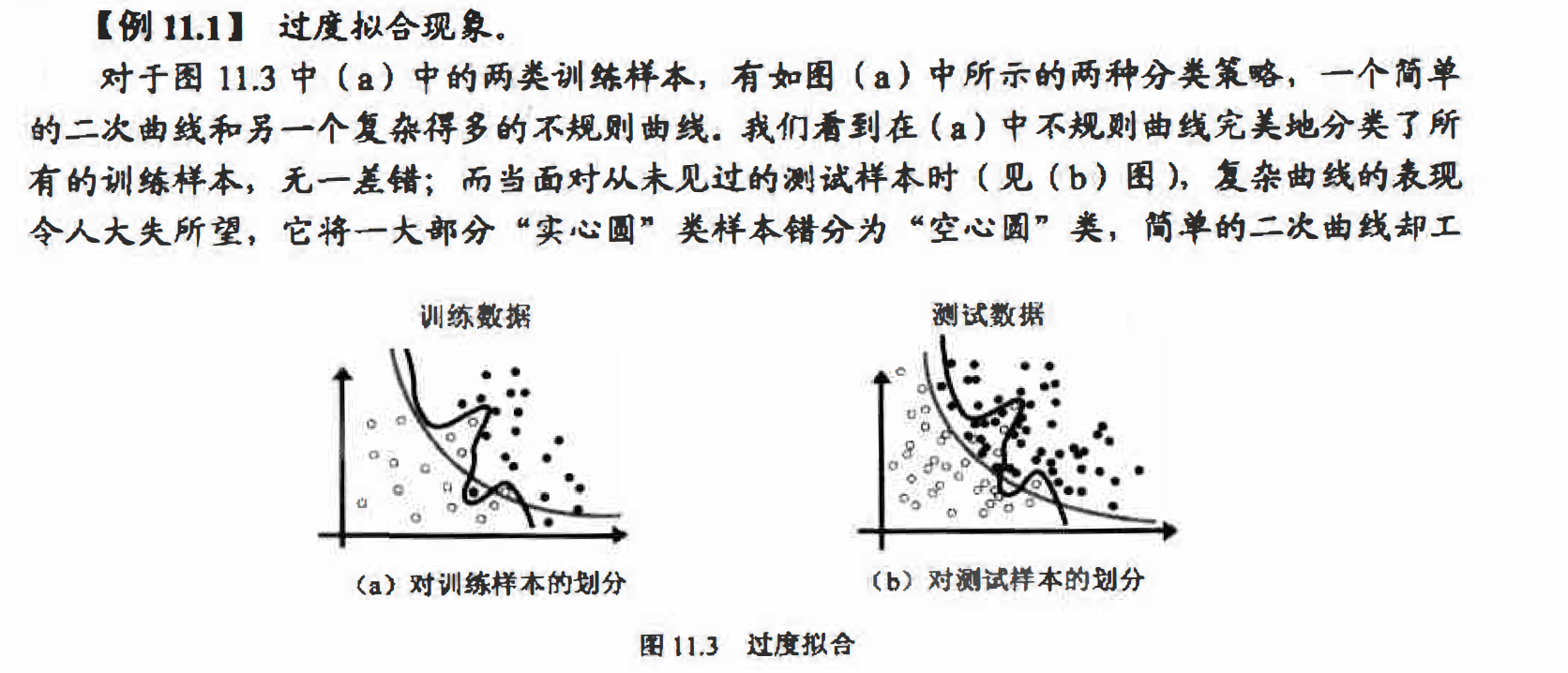作得相当好．究其原因，主要是过度复杂的决策边界不能够对新数据进行很好地归纳（泛化一般化），它们过于倾向对训练数据的正确划分（复杂的形式正好为它们完美地拟合训练数据 创造了条件），而不能够对真正的数据模型进行很好地分类． 这个问题称为过度拟合(overfit)简单的决策边界对训练数据不够理想， 但是对新数据却往往能够较好地归纳 ．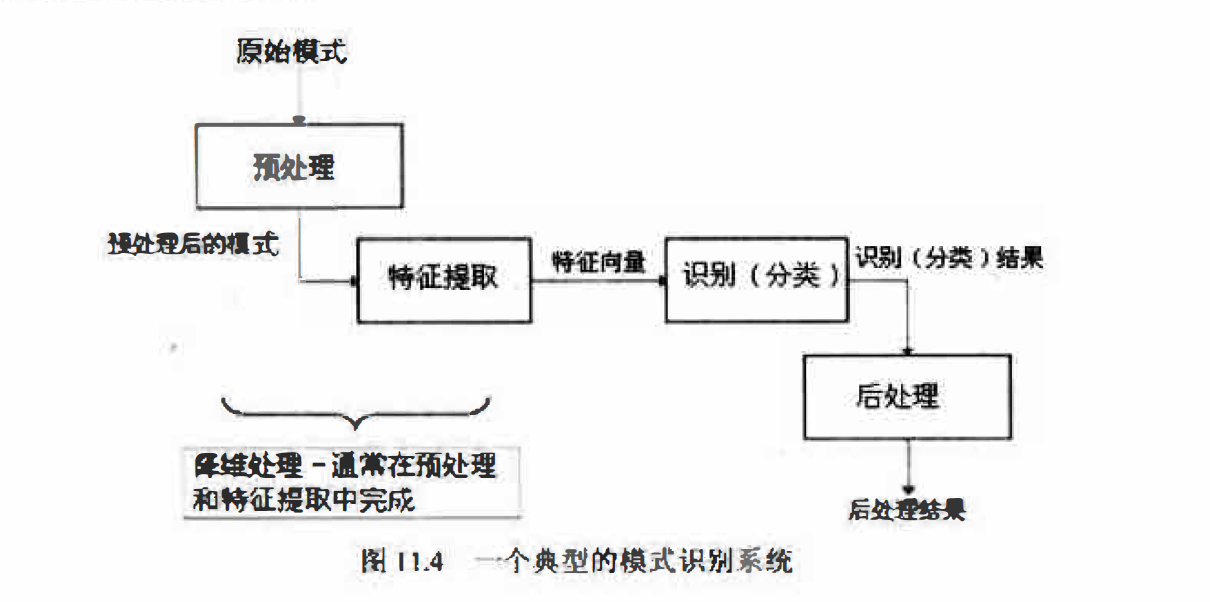#### 训练学习方法分类

• 教师指导的学习：又称为有监督学习。是指在训练样本集中的每个输入样本类别均已知的情况下进行学习，也就是使用训练模式和相应的类别标记一起来” 教授“ 分类器．日常生活中有监督学习的一个例子是教孩子识字，教师将字本身（样本）和具体是什么字（类别） 一起教给孩子。
• 无教师指导的学习：又称为无监督学习．是指在样本中没有相应的类别信息的情况下，系统对输入样本自动形成“自然的” 组织或簇(cluster). 如“ 聚类算法” 就是一种典型的无监督学习.
• 加强学习：又称为基于评价的学习．在加强学习中，并不把类别信息直接提供给分类器，而是让分类器自己根据输入样本计算输出类别，将它与已知的类别标记进行比较,判断对已知训练模式的分类是否正确，从而辅助分类器的学习。日常生活中加强学习的一个例子是提供正确答案的考试讲评，这里考生就相当于分类器，他们先是进行考试（分类）， 然后根据教师提供的标准答案来改善知识体系（分类器模型）．

#### 模式识别方法分类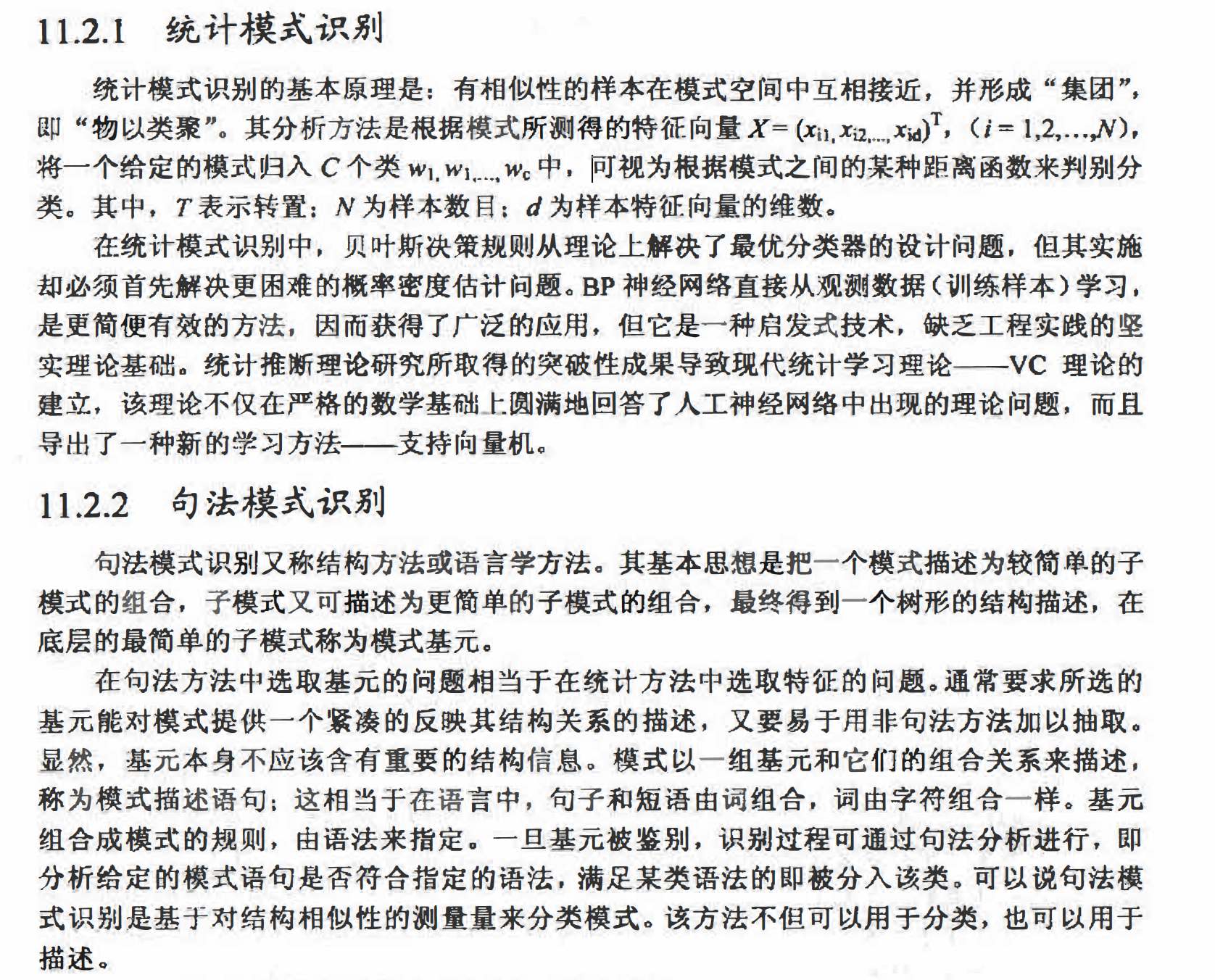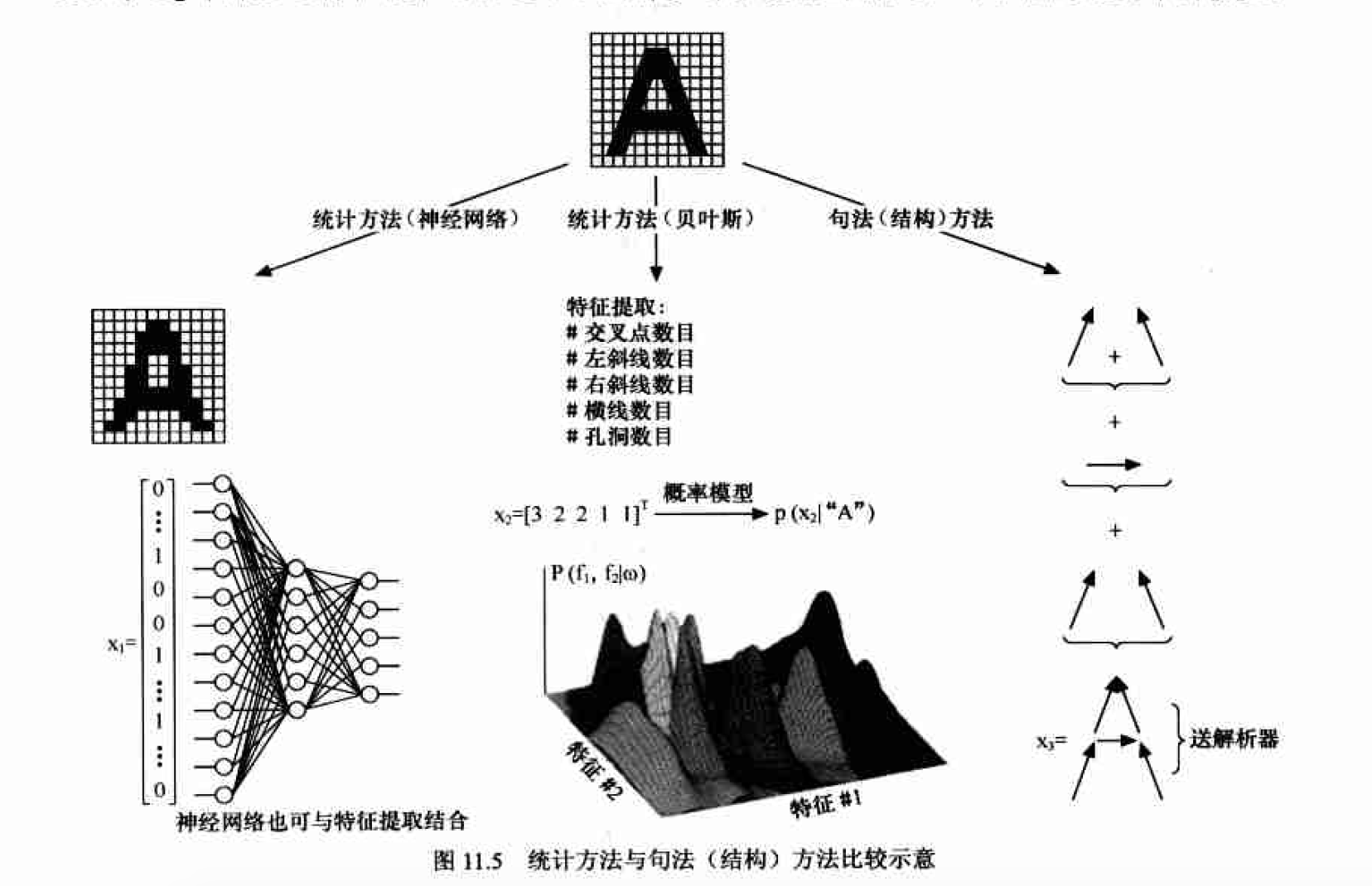### 最小距离分类器和模板匹配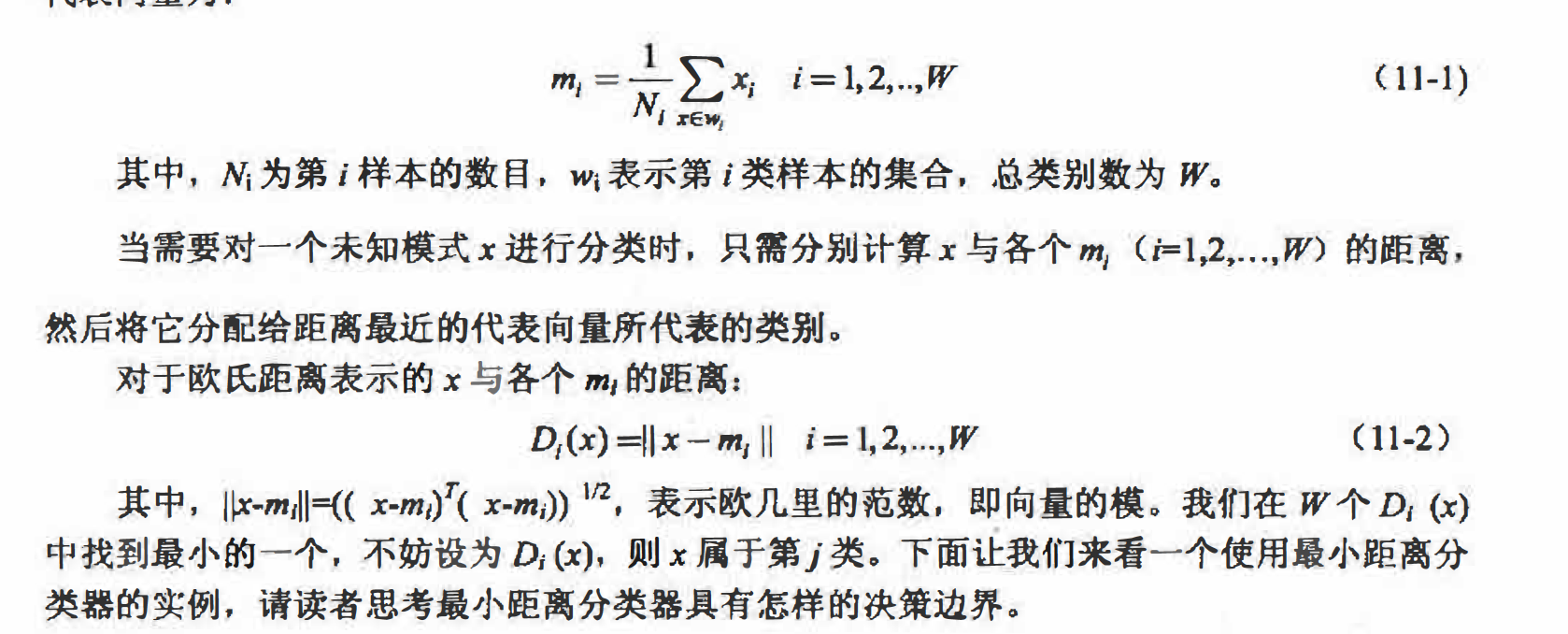% 例11.3 利用最小距离分类器分类３种鸢尾属植物

% 每类的前40个样本用于生成代表该类的模板，后10个作为独立的测试样本
m1 = mean( meas(1:40, :) ); %第1类的前40个样本的平均向量
m2 = mean( meas(51:90, :) ); %第2类的前40个样本的平均向量
m3 = mean( meas(101:140, :) ); %第3类的前40个样本的平均向量

% 测试样本集
Test = [meas(41:50, :); meas(91:100, :); meas(141:150, :)];
% 测试样本集对应的类别标签
classLabel(1:10) = 1;
classLabel(11:20) = 2;
classLabel(21:30) = 3;

% 利用最小距离分类器分类测试样本
class = zeros(1, 30); %类标签
for ii = 1:size(Test, 1)
d(1) = norm(Test(ii, :) - m1); %与第１类的距离
d(2) = norm(Test(ii, :) - m2); %与第２类的距离
d(3) = norm(Test(ii, :) - m3); %与第３类的距离

[minVal class(ii)] = min(d); %计算最小距离并将距离样本最短的类赋给类标签数组 class
end

% 测试最小距离分类器的识别率
nErr = sum(class ~= classLabel);
rate = 1 - nErr / length(class);
strOut = ['识别率为', num2str(rate*100), '%']


#### 基于相关的模板匹配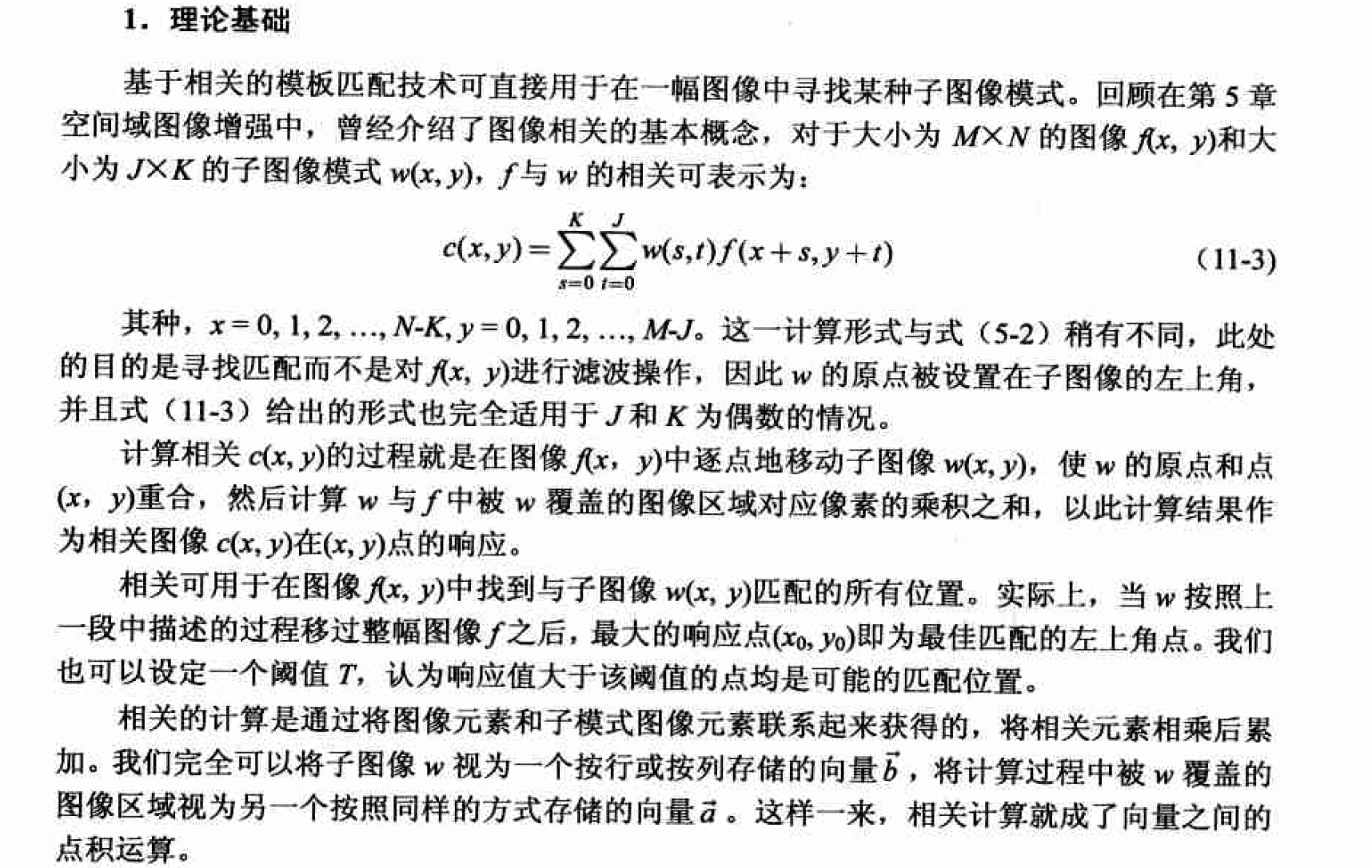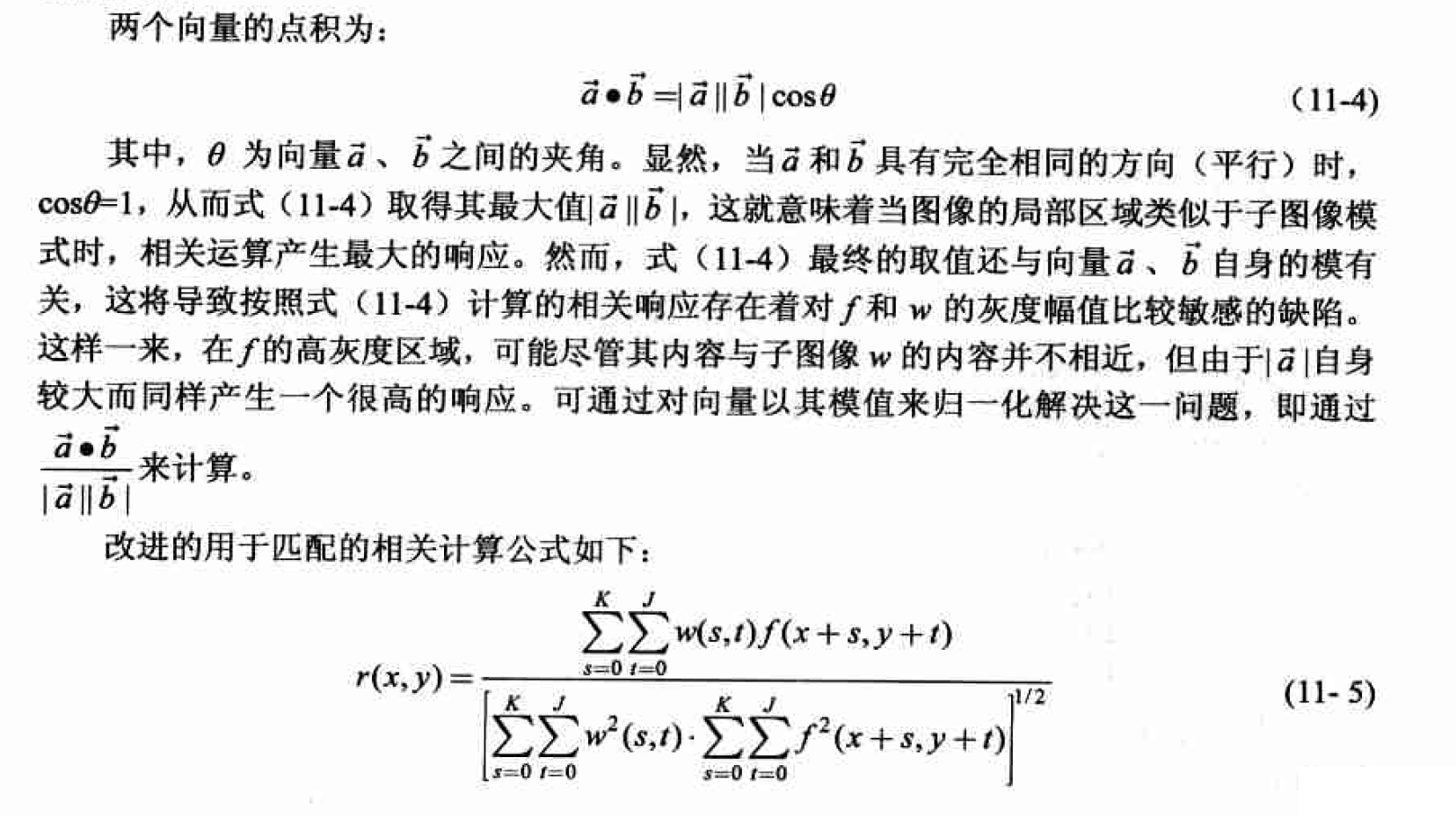matlab实现

function Icorr = imcorr(I, w)
% function Icorr = imcorr(I, w, )
% 计算图像 I 与子模式 w 的相关响应，并提示最大的响应位置
%
% Input：I - 原始图像
%        w - 子图像
%
% Output：Icorr - 响应图像

[m, n] = size(I);
[m0, n0] = size(w);

Icorr = zeros(m-m0+1, n-n0+1); %为响应图像分配空间

vecW = double( w(:) ); %按列存储为向量
normW = norm(vecW); %模式图像对应向量的模

for ii = 1:m-m0+1
for jj = 1:n-n0+1
subMat = I(ii:ii+m0-1, jj:jj+n0-1);
vec = double( subMat(:) ); %按列存储为向量
Icorr(ii, jj) = vec' * vecW / (norm(vec)*normW+eps); %计算当前位置的相关
end
end

% 找到最大响相应位置
[iMaxRes, jMaxRes] = find(Icorr == max( Icorr(:) ) );
figure, imshow(I);
hold on
for ii = 1:length(iMaxRes)
plot(jMaxRes(ii), iMaxRes(ii), 'w*');
plot([jMaxRes(ii), jMaxRes(ii)+n0-1], [iMaxRes(ii), iMaxRes(ii)], 'w-' );
plot([jMaxRes(ii)+n0-1, jMaxRes(ii)+n0-1], [iMaxRes(ii), iMaxRes(ii)+m0-1], 'w-' );
plot([jMaxRes(ii), jMaxRes(ii)+n0-1], [iMaxRes(ii)+m0-1, iMaxRes(ii)+m0-1], 'w-' );
plot([jMaxRes(ii), jMaxRes(ii)], [iMaxRes(ii), iMaxRes(ii)+m0-1], 'w-' );
end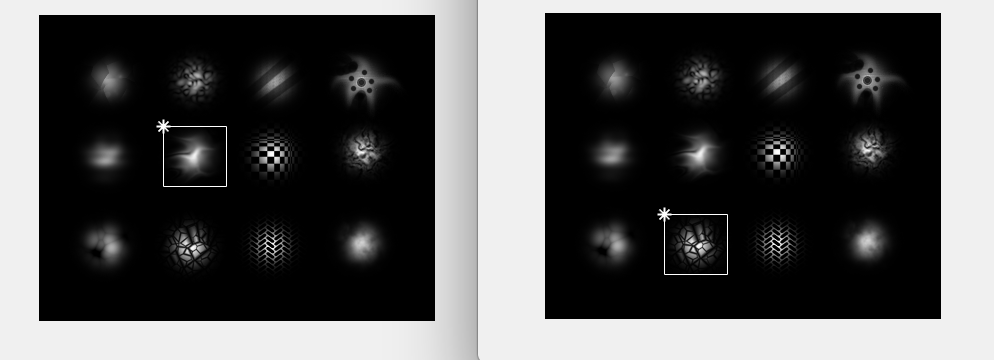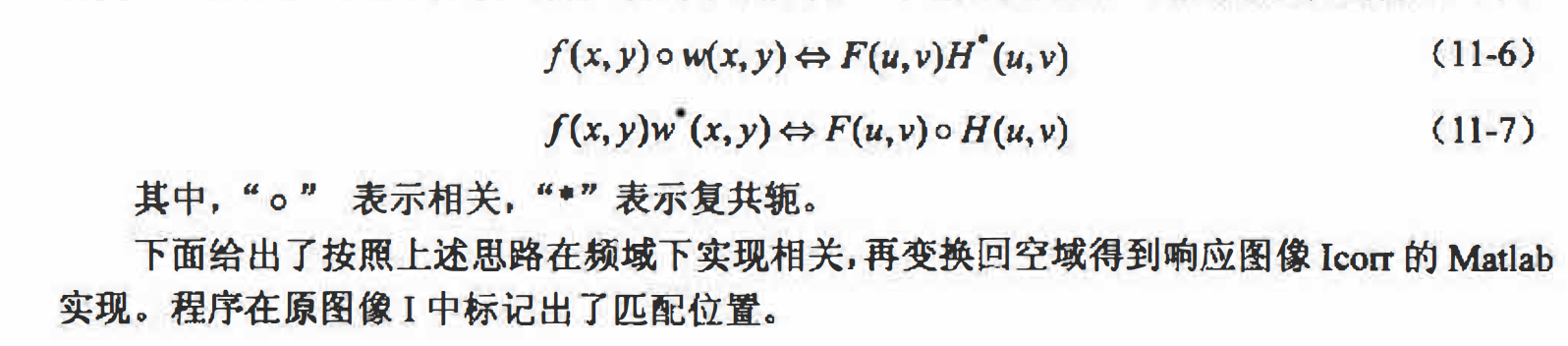function Icorr = dftcorr(I, w)
% function Icorr = dftcorr(I, w)
% 在频域下计算图像 I 与子模式 w 的相关响应，并提示最大的响应位置
%
% Input：I - 原始图像
%        w - 子图像
%
% Output：Icorr - 响应图像
I = double(I);
[m n] = size(I);
[m0 n0] = size(w);
F = fft2(I);
w = conj(fft2(w, m, n)); %w 频谱的共轭
Ffilt = w .* F; %频域滤波结果
Icorr = real(ifft2(Ffilt)); %反变换回空域

% 找到最响相应位置
[iMaxRes, jMaxRes] = find(Icorr == max( Icorr(:) ) );
figure, imshow(I, []);
hold on
for ii = 1:length(iMaxRes)
plot(jMaxRes(ii), iMaxRes(ii), 'w*');
plot([jMaxRes(ii), jMaxRes(ii)+n0-1], [iMaxRes(ii), iMaxRes(ii)], 'w-' );
plot([jMaxRes(ii)+n0-1, jMaxRes(ii)+n0-1], [iMaxRes(ii), iMaxRes(ii)+m0-1], 'w-' );
plot([jMaxRes(ii), jMaxRes(ii)+n0-1], [iMaxRes(ii)+m0-1, iMaxRes(ii)+m0-1], 'w-' );
plot([jMaxRes(ii), jMaxRes(ii)], [iMaxRes(ii), iMaxRes(ii)+m0-1], 'w-' );
end